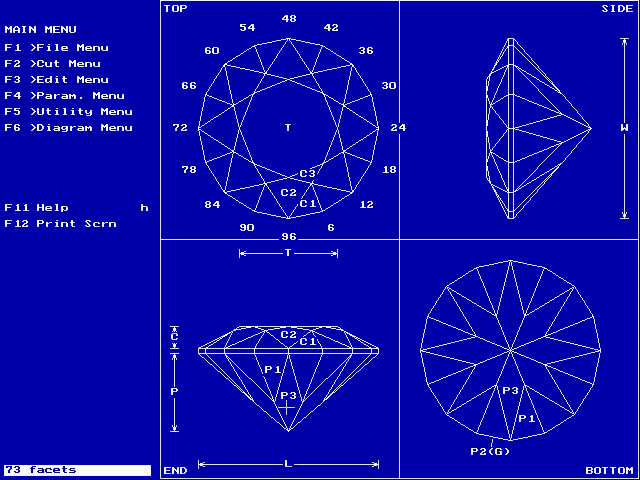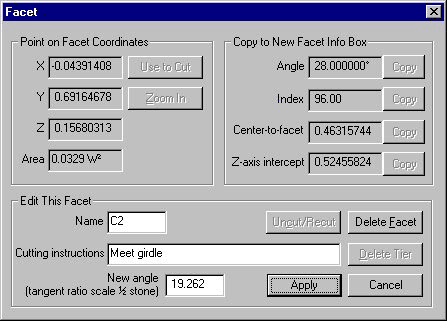### What to do with the results of BOG

Back to Main Page

BOG helps you select the optimal pavillion and crown angles for your gem. It does not, however, alter the input GemCAD files in any way. In fact, for safety, BOG works with a copy of the file. You will have to adjust the gem angles manually using the tangent ratio method.

To illustrate the procedure, we will apply the optimized angles from the QuickStart Tutorial to the BOGTUT.GEM file. In the tutorial, we optimized the face-up ISO brightness of the gem, deriving new pavillion and crown angles:

 Facet Original Optimized Pavillion (P3) 41 40.477 Crown (C2) 28 19.262

Note that these are not necessarily the best angles for this stone. Follow the full tutorial sequence to learn more about additional optimization targets. For now, however, we will apply these angles.

There are a number of ways of applying the tangent ratio method (and don't worry if you are unfamiliar with GemCAD or tangent ratios. The process is very simple!)

### Important Note

There are two versions of GemCAD, available at http://www.gemcad.com. The original DOS version has been updated for Windows. Performing a tangent ratio calculation is easy in both versions, as explained below.

### Manual Tangent Ratio Conversion

The tangent ratio method adjusts a gem's angles without altering its plan view, i.e. the facet outlines when seen from above. Mathematically, each angle in the gem design is replaced by a new value given by:

NewAngle = InvTan (Tan(OldAngle) * TR)

where Tan and InvTan refer to the trigonometric tangent function, and TR is a scale factor known as the "tangent ratio":

TR = Tan(NewRef) / Tan(OldRef)

where NewRef and OldRef are the reference angles in the design - the ones you specified within BOG.

For example, in the QuickStart Tutorial, we used the crown main facets at 28 degrees as the reference (OldRef) and derived an optimized angle of 19.262 degrees (NewRef). See the table above. The tangent ratio for the BOGTUT.GEM crown is then:

TR = Tan(19.262) / Tan(28.0) = 0.657

All the other crown angles use this TR. For example, the star facets at 13 degrees become:

NewAngle = InvTan (Tan(13.0) * TR) = 8.62 degrees

Any simple hand calculator with trigonometric functions will allow you to perform these calculations. Don't forget to use the appropriate TR for the pavillion! It will not, in general, be the same TR as for the crown. For BOGTUT.GEM, the pavillion TR is:

TR = Tan(40.477) / Tan(41.0) = 0.982

Note that the TR value is also the scale factor for the height of the stone. In the example shown here, the new crown will be 65.7% as high as the original. You can use this fact to adjust the limits of angles that BOG explores. For example, internal flaws or the amount of remaining facet rough may restrict the height of the crown. The ratio of usable material to the amount needed is exactly equal to TR. You can then calculate a maximum usable NewRef for a given crown angle OldRef in the design. Enter this new angle as the Max crown value in the Specify Angles and Materials dialog box.

Top of page

### Online Tangent Ratio Conversion

You can easily adjust the gemstone design using online tangent ratio converters. I like the one provided by Bob Keller at:

http://www.rockhounds.com/rockshop/gem_designs/tangent_ratio_form.html (Actual calculator)

For example, in the QuickStart Tutorial, we used the crown main facets at 28 degrees as the reference and derived an optimized angle of 19.262 degrees. See the table above. Simply enter these values into the appropriate boxes, add the remaining crown angles, then click Submit for Processing:The online calculator will report the new values in a few seconds. As a double check, I usually enter the Original Reference Angle (here 28 degrees) as one of the Angles, and check that the answer equals the New Reference Angle.

Top of page

Double-click the GemCAD icon to begin the program. If this is the first time you have run GemCAD, you may have to answer some questions for the initial configuration (see the GemCAD documentation). Use the O (uppercase o) command or navigate the menus to open the BOGTUT.GEM file. You should see the following:This is the original, unoptimized gem. You can see the cutting instructions using the l command (or Diagram Menu : List to scrn). Note that during the QuickStart Tutorial, we selected pavillion facet P3 and crown facet C2 as the "old" angles to be optimized. Press esc to return to the drawing.

#### Step 2 - Scale the Gem using Tangent-Ratio

Place the cursor on P3, the pavillion facet to be adjusted and press T. Alternatively, select Edit Menu: Tan Ratio then click on facet P3. Gemcad should prompt you with: Angle was 41.00. New Angle?

If you see a different angle in the prompt, make sure that you have the cursor in the correct location (see figure above), and double check the cutting instructions with the l key.

Enter the optimized pavillion angle (40.477) and hit return. GemCAD will redraw the gem with the appropriately scaled pavillion. The drawing may look very similar, but you can confirm the change by again listing the cutting instructions on the screen.

Repeat this procedure for the crown, changing C2 from 28 to 19.262. If you watch carefully, it should be easy to see that the new crown is considerably flatter.

#### Step 3 - Save the Gem and Print Cutting Instructions

At this point, you have scaled both the pavillion and crown angles to their optimized values. You can save the gem under a new name (S or File Menu: Save as) or overwrite BOGTUT.GEM (s or File Menu: Save). Quit the GemCAD program using the Q command or File Menu: Quit.

The modified gem should now be in the specified GemCAD file. Print it out in the usual way using GemPrint.exe or Windows GemCAD.

Top of page

Double-click the GemCAD icon to begin the program. Use the Open command in the File menu to load BOGTUT.GEM. You should see the following:#### Step 2 - Scale the Gem using Tangent-Ratio

Place the cursor on C2, the crown facet to be adjusted (see above) and left click. A dialog box should appear:Enter the New angle (here 19.262) in the appropriate box at the bottom. Click the Apply button to scale the crown. Use the identical procedure to adjust the pavillion.

#### Step 3 - Save the Gem and Print Cutting Instructions

At this point, you should have scaled both the pavillion and crown angles to their optimized values. You can save the gem under a new name (File Menu: Save as) or overwrite BOGTUT.GEM (File Menu: Save). You can now print it out the design in the usual way (File Menu:Print).

Back to Main Page.